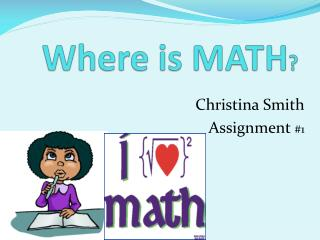Download PresentationWhere is MATH ?

# Where is MATH ?

Download Presentation## Where is MATH ?

- - - - - - - - - - - - - - - - - - - - - - - - - - - E N D - - - - - - - - - - - - - - - - - - - - - - - - - - -
##### Presentation Transcript

1. Where is MATH? Christina Smith Assignment #1

2. Essential Question • What are some things you do throughout the day that involve math?

3. Unit Questions • What games do you play that involve math? • Why is it important to know math?

4. TEKS

5. TEKS 111.17 Mathematics, Grade 5 • (5.1) Number, operation, and quantitative reasoning. The student uses place value to represent whole numbers and decimals. The student is expected to: • (B) use place value to read, write, compare, and order decimals through the thousandths place.

6. TEKS 111.17 Mathematics, Grade 5 • (5.12) Probability and statistics. The student describes and predicts the results of a probability experiment. The student is expected to: • (B) use experimental results to make predictions • (C) list all possible outcomes of a probability experiment such as tossing a coin

7. TEKS 111.17 Mathematics, Grade 5 • (5.14) Underlying processes and mathematical tools. The student applies Grade 5 mathematics to solve problems connected to every day experiences and activities in and outside of school. The student is expected to: • (A) identify the mathematics in everyday situations • (B) solve problems that incorporate understanding the problem, making a plan, carrying out the plan, and evaluating the solution for reasonableness • (D) use tools such as real objects, manipulative, and technology to solve problems.

8. Bloom’s Taxonomy • With the “Money Problems” activity, students will be able to cover all levels of Bloom’s Taxonomy because the activity involves money which students already know about. The activity is about balancing check books, so the students are going to have to understand how balancing a check book goes. With the activity they play, they’ll be able to apply it to the activity.

9. Bloom’s Taxonomy Cont… • In the “Dice Probabilities” activity, the students will be using Bloom’s Taxonomy by applying it to the game being played. The students will be able to collect the data, and chart the information. Through illustration they will compare their predictions with the data they recorded.

10. ACTIVITIES

11. MONEY PROBLEMS • Try an experiment comparing the same balance in different types of bank accounts. How much better is a savings account than a checking account? What difference does the interest rate make? Which is better, an account that earns compound or simple interest? Can you compare the short and long term costs of borrowing money compared to saving the cash for a purchase?

12. Dice Probabilities • The purpose of this project is to test the probabilities of rolling certain combinations of dice in roll-playing games. Are you more likely to roll a sum of at least 18 with 3 ten-sided dice or 5 six-sided dice?

13. REFERENCES • http://www.sciencebuddies.org • http://mathforum.org/dr.math/faq/faq.doubling.pennies.html • http://library.thinkquest.org/11506/learn.html